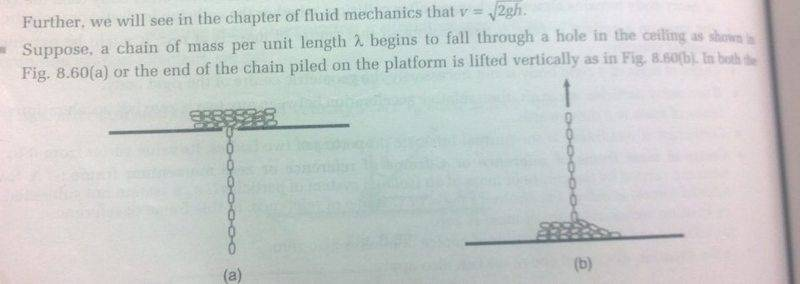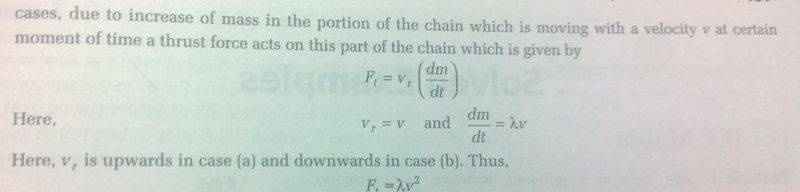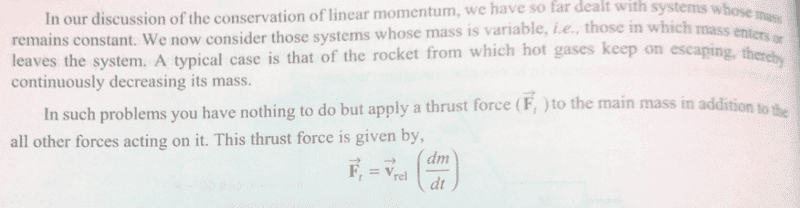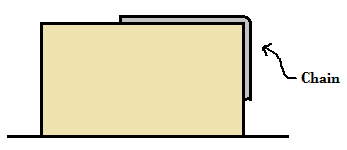# Work energy theorem problem

• Titan97

Gold Member

## Homework Statement

A uniform chain of mass m and length l overhangs on a smooth table with two third part lying on the table. Find the kinetic energy of the chain after it slips off the table.

## Homework Equations

F=ma
Total work done by all forces = change in kinetic energy

## The Attempt at a Solution

I got the answer to this question (##\frac{4}{9}mgl##) by finding the change in potential energy. I also got the same answer using work-energy theorem. I tried using a third method by using Newton's laws to find the acceleration of the chain at any moment. Let 'n'th part of chain be on the table (##n\le 1##).
For the part of chain on the table, ##N=nmg## where N is the normal reaction.
Along horizontal, tension T acts. ##T=nma##
But here is my doubt. Yesterday, I learned about thrust force from my physics textbook. This is what's written:In this case, the mass of the chain on the table decreases. So a thrust force acts on that part of chain. Does this mean my previous equation are wrong?
(here is another pic:)

Last edited:

I've never heard of "thrust force" in such a situation and you link cannot be opened.

I have edited the post. If you can't see the images, then i will type it. Please reply so I can do something about it. (I can see the images)

First, there is an important difference between a chain sliding off the side of a smooth table and, on the other hand, either falling through a smooth hole or being lifted from a pile.
When a chain or rope slides off the side of a table it will acquire a horizontal velocity component. Work will be conserved, but the shape will not be a vertical line. At the moment when the trailing end leaves the table the location of the mass centre is very hard to determine, so the question, taken strictly, is extremely hard to answer.
When it slides througn a smooth hole, no horizontal velocity is acquired. However, you can no longer assume work is conserved. Instead, you should use conservation of momentum.

A thrust is a force (so thrust force is tautologous, it's just 'thrust'). Specifically, it is the reaction force to a force which is accelerating a mass. I don't believe it applies in this context. The force of tension in the suspended chain is different at different points of the chain. The equation shown applies to the tension at the top. It tells you what tension is needed to accelerate the links as they leave the table. The reaction force would be the corresponding gravitational force that the chain exerts on the Earth, which is conceptually distinct. Also, that force has a component corresponding to the acceleration of the part of the chain which has already left the table.

•Titan97
Why can't i use work energy theorem for a chain sliding down a smooth hole? Also, why can't i apply thrust force in the case of a chain sliding of a table? When the chain falls through a smooth hole, its vertical velocity increase. So why can't the change in kinetic energy be equal to change in potential energy? For a chain falling through a smooth hole, the mass of the chain that hasn't piled up decreases. The change in momentum due to change in mass is taken as thrust. (?)
I need your help in deriving the expression for thrust in case of a chain sliding through a smooth hole assuming that it does not pile up as it hits the floor.
@haruspex I have asked you 4 questions. I don't require full solution/answer. I just need some help. I don't know if PF allows someone to do so. But please help me learn the concepts.

Why can't i use work energy theorem for a chain sliding down a smooth hole? Also, why can't i apply thrust force in the case of a chain sliding of a table? When the chain falls through a smooth hole, its vertical velocity increase. So why can't the change in kinetic energy be equal to change in potential energy? For a chain falling through a smooth hole, the mass of the chain that hasn't piled up decreases. The change in momentum due to change in mass is taken as thrust. (?)
I need your help in deriving the expression for thrust in case of a chain sliding through a smooth hole assuming that it does not pile up as it hits the floor.
@haruspex I have asked you 4 questions. I don't require full solution/answer. I just need some help. I don't know if PF allows someone to do so. But please help me learn the concepts.
This hole problem fools some of the experts, so don't be surprised you are struggling with it.
It is discussed in section 1 of https://www.physicsforums.com/insights/frequently-made-errors-mechanics-momentum-impacts/.
You should doubt conservation of work when there is an apparently infinite acceleration. The portion of chain on the table is considered to be at rest, but as the next bit heads into the hole it somehow gets up to the speed of the falling portion instantly.
The clincher is that when you apply conservation of momentum (or, equivalently, the analysis you quoted in the OP) you get a different answer.

For the chain off the side of the table, I should have broken it further into two cases. The chain may be laid out straight perpendicular to the table edge (so the whole chain accelerates together) or in a pile near the edge.
Either way, each bit leaves the table horizontally. The weight of the suspended chain cannot pull that directly vertical, it will retain some horizontal movement. In consequence the descending chain will describe an interesting arc through the air. The maths of that are very complicated. At the point where the trailing end of the chain reaches the edge of the table, the height of the mass centre of the chain is therefore very hard to determine, and without that we do not know the loss in PE.

•Titan97
This is the given situation:Correct me if I am wrong.
As the chain begins to slide, "The portion of chain on the table is considered to be at rest". It is pulled by the vertical part and begins motion instantaneously. So I can't use work-energy theorem?
I am not able to understand why "The mistake is the assumption that work is conserved even though, as each element of string is picked up in the departing thread, it apparently accelerates instantaneously from rest to speed v."
In the above case, the gain in kinetic energy=loss in potential energy. I can find the potential energy of the chain by using integration. (taking the top of the table as zero potential energy level).
So whatever answer I get is wrong?

"The portion of chain on the table is considered to be at rest". It is pulled by the vertical part and begins motion instantaneously. So I can't use work-energy theorem?
I am not able to understand why "The mistake is the assumption that work is conserved even though, as each element of string is picked up in the departing thread, it apparently accelerates instantaneously from rest to speed v."
No, you're mixing up the two cases. The comments of mine that you quote above were for the chain falling through a hole (or being pulled straight up) from a loose pile.
Your diagram in post #7 is the other case, the chain falling off the side of a table. Also, it shows the chain as laid out straight on the table. In this case, the whole chain accelerates together and is taut at all times. In consequence, there are no seemingly infinite accelerations and work is conserved. The difficulty now is that the diagram shows the hanging part only as it would be right at the start. Once the system is moving, it will not hang straight but arc away from the table. This makes it hard to know how high the mass centre is at any given time, so hard to figure out how much PE has been lost.

•Titan97
Same problem can also be found in the book by I.E.Irodov. Both have assumed that table has a height greater than length of chain. Hence the center of mass is at a distance y/2 from top where y is the length of the overhanging part of chain. Also, they might have assumed that the chain sticks to the table and does not arc from the table.
Now, why can't I use work energy theorem when there is infinite acceleration?

Same problem can also be found in the book by I.E.Irodov. Both have assumed that table has a height greater than length of chain. Hence the center of mass is at a distance y/2 from top where y is the length of the overhanging part of chain. Also, they might have assumed that the chain sticks to the table and does not arc from the table.
In order to get y/2, you will neeed to make such an assumption. But it should not be an assumption. The question statement should give you some reason to take it as the case. Moreover, the sticking to the side of the table needs to be achieved in such a way that work is conserved. That is, the lost horizontal KE has to be converted into vertical KE. That is why some formulations mention a smooth tube ducting the chain from horizontal to vertical.
Now, why can't I use work energy theorem when there is infinite acceleration?
Edit: to clarify, the rest of this post is in relation to the case where the chain falls through a hole in the table.

It's not that the acceleration can really be infinite. It's just that in problems where there are very sudden acelerations, impacts, it is often the case that work will not be conserved. In general, it is safer to assume that it is not. If there is enough information to solve the problem without assuming it is conserved then that is the right way to go.
In this specific case, there is enough information, the answer shows that work is not conserved, and the reasons why it will not be conserved are discussed at the page I linked to ("where did the work go?").

Last edited:
•Titan97